# nlarx

Estimate parameters of nonlinear ARX model

## Syntax

``sys = nlarx(data,orders)``
``sys = nlarx(data,regressors)``
``sys = nlarx(___,output_fcn)``
``sys = nlarx(data,linmodel)``
``sys = nlarx(data,linmodel,output_fcn) ``
``sys = nlarx(data,sys0)``
``sys = nlarx(___,Options)``

## Description

### Specify Regressors

example

````sys = nlarx(data,orders)` estimates a nonlinear ARX model to fit the given estimation data using the specified ARX model orders and the default wavelet network output function. Use this syntax when you extend an ARX linear model, or when you use only regressors that are linear with consecutive lags.```

example

````sys = nlarx(data,regressors)` estimates a nonlinear ARX model using the specified regressor set `regressors`. Use this syntax when you have linear regressors that have non-consecutive lags, or when you also have any combination of polynomial regressors, periodic regressors, and custom regressors.```

example

````sys = nlarx(___,output_fcn)` specifies the output function that maps the regressors to the model output. You can use this syntax with any of the previous input argument combinations.```

### Specify Linear Model

example

````sys = nlarx(data,linmodel)` uses a linear ARX model `linmodel` to specify the model orders and the initial values of the linear coefficients of the model. Use this syntax when you want to create a nonlinear ARX model as an extension of, or an improvement upon, an existing linear model. When you use this syntax, the software initializes the offset value to `0`. In some cases, you can improve the estimation results by overriding this initialization with the command `sys.OutputFcn.Offset.Value = NaN`.```

example

````sys = nlarx(data,linmodel,output_fcn) ` specifies the output function to use for model estimation.```

### Refine Existing Model

example

````sys = nlarx(data,sys0)` estimates or refines the parameters of the nonlinear ARX model `sys0`.Use this syntax to:Estimate the parameters of a model previously created using the `idnlarx` constructor. Prior to estimation, you can configure the model properties using dot notation.Update the parameters of a previously estimated model to improve the fit to the estimation data. In this case, the estimation algorithm uses the parameters of `sys0` as initial guesses.```

### Specify Options

example

````sys = nlarx(___,Options)` specifies additional configuration options for the model estimation.```

## Examples

collapse all

`load twotankdata;`

Create an `iddata` object from the estimation data with a sample time of 0.2 seconds.

```Ts = 0.2; z = iddata(y,u,Ts);```

Estimate the nonlinear ARX model using ARX model orders to specify the regressors.

`sysNL = nlarx(z,[4 4 1])`
```sysNL = Nonlinear ARX model with 1 output and 1 input Inputs: u1 Outputs: y1 Regressors: Linear regressors in variables y1, u1 List of all regressors Output function: Wavelet network with 11 units Sample time: 0.2 seconds Status: Estimated using NLARX on time domain data "z". Fit to estimation data: 96.84% (prediction focus) FPE: 3.482e-05, MSE: 3.431e-05 ```

`sys` uses the default `idWaveletNetwork` function as the output function.

For comparison, compute a linear ARX model with the same model orders.

`sysL = arx(z,[4 4 1]);`

Compare the model outputs with the original data.

`compare(z,sysNL,sysL)`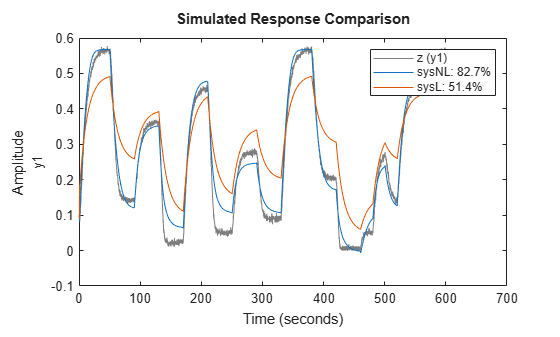The nonlinear model has a much better fit to the data than the linear model.

Specify a linear regressor that is equivalent to an ARX model order matrix of `[4 4 1]`.

An order matrix of `[4 4 1]` specifies that both input and output regressor sets contain four regressors with lags ranging from 1 to 4. For example, ${\mathit{u}}_{1}\left(\mathit{t}-2\right)$ represents the second input regressor.

Specify the output and input names.

```output_name = 'y1'; input_name = 'u1'; names = {output_name,input_name};```

Specify the output and input lags.

```output_lag = [1 2 3 4]; input_lag = [1 2 3 4]; lags = {output_lag,input_lag};```

Create the linear regressor object.

`lreg = linearRegressor(names,lags)`
```lreg = Linear regressors in variables y1, u1 Variables: {'y1' 'u1'} Lags: {[1 2 3 4] [1 2 3 4]} UseAbsolute: [0 0] TimeVariable: 't' Regressors described by this set ```

Load the estimation data and create an iddata object.

```load twotankdata z = iddata(y,u,0.2);```

Estimate the nonlinear ARX model.

`sys = nlarx(z,lreg)`
```sys = Nonlinear ARX model with 1 output and 1 input Inputs: u1 Outputs: y1 Regressors: Linear regressors in variables y1, u1 List of all regressors Output function: Wavelet network with 11 units Sample time: 0.2 seconds Status: Estimated using NLARX on time domain data "z". Fit to estimation data: 96.84% (prediction focus) FPE: 3.482e-05, MSE: 3.431e-05 ```

View the regressors

`getreg(sys)`
```ans = 8x1 cell {'y1(t-1)'} {'y1(t-2)'} {'y1(t-3)'} {'y1(t-4)'} {'u1(t-1)'} {'u1(t-2)'} {'u1(t-3)'} {'u1(t-4)'} ```

Compare the model output to the estimation data.

`compare(z,sys)`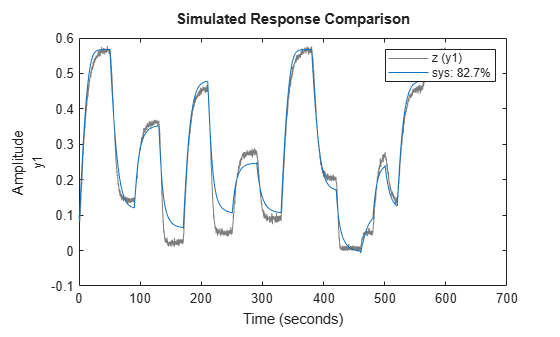Create time and data arrays.

```dt = 0.01; t = 0:dt:10; y = 10*sin(2*pi*t)+rand(size(t));```

Create an `iddata` object with no input signal specified.

`z = iddata(y',[],dt);`

Estimate the nonlinear ARX model.

`sys = nlarx(z,2)`
```sys = Nonlinear time series model Outputs: y1 Regressors: Linear regressors in variables y1 List of all regressors Output function: Wavelet network with 8 units Sample time: 0.01 seconds Status: Estimated using NLARX on time domain data "z". Fit to estimation data: 92.92% (prediction focus) FPE: 0.2568, MSE: 0.2507 ```

Estimate a nonlinear ARX model that uses the mapping function `idSigmoidNetwork` as its output function.

Load the data and divide it into the estimation and validation data sets `ze` and `zv`.

```load twotankdata.mat u y z = iddata(y,u,'Ts',0.2); ze = z(1:1500); zv = z(1501:end);```

Configure the `idSigmoidNetwork` mapping function. Fix the offset to 0.2 and the number of units to 15.

```s = idSigmoidNetwork; s.Offset.Value = 0.2; s. NonlinearFcn.NumberOfUnits = 15;```

Create a linear model regressor specification that contains four output regressors and five input regressors.

`reg1 = linearRegressor({'y1','u1'},{1:4,0:4});`

Create a polynomial model regressor specification that contains the squares of two input terms and three output terms.

`reg2 = polynomialRegressor({'y1','u1'},{1:2,0:2},2);`

Set estimation options for the search method and maximum number of iterations.

```opt = nlarxOptions('SearchMethod','fmincon')'; opt.SearchOptions.MaxIterations = 40;```

Estimate the nonlinear ARX model.

`sys = nlarx(ze,[reg1;reg2],s,opt);`

Validate `sys` by comparing the simulated model response to the validation data set.

`compare(zv,sys)`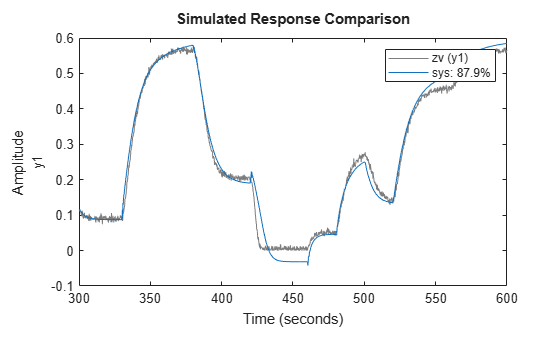Estimate a linear model and improve the model by adding a `treepartition` output function.

`load throttledata ThrottleData`

Estimate a linear ARX model `linsys` with orders `[2 2 1]`.

`linsys = arx(ThrottleData,[2 2 1]);`

Create an `idnlarx` template model that uses `linsys` and specifies `sigmoidnet` as the output function.

`sys0 = idnlarx(linsys,idTreePartition);`

Fix the linear component of `sys0` so that during estimation, the linear portion of `sys0` remains identical to `linsys`. Set the offset component value to `NaN`.

```sys0.OutputFcn.LinearFcn.Free = false; sys0.OutputFcn.Offset.Value = NaN;```

Estimate the free parameters of `sys0`, which are the nonlinear-function parameters and the offset.

`sys = nlarx(ThrottleData,sys0);`

Compare the fit accuracies for the linear and nonlinear models.

`compare(ThrottleData,linsys,sys)`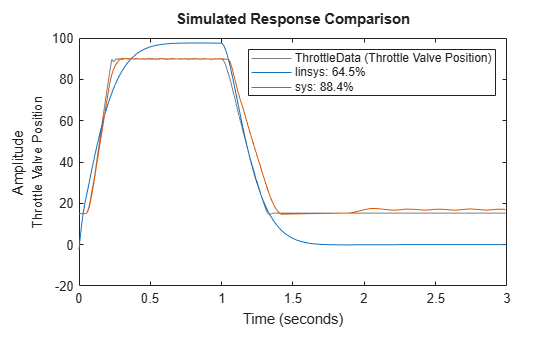Generating a custom network mapping object requires the definition of a user-defined unit function.

Define the unit function and save it as `gaussunit.m`.

```function [f,g,a] = gaussunit(x) % Custom unit function nonlinearity. % % Copyright 2015 The MathWorks, Inc. f = exp(-x.*x); if nargout>1 g = -2*x.*f; a = 0.2; end ```

Create a custom network mapping object using a handle to the `gaussunit` function.

```H = @gaussunit; CNet = idCustomNetwork(H); ```

```load iddata1 ```

Estimate a nonlinear ARX model using the custom network.

```sys = nlarx(z1,[1 2 1],CNet) ```
```sys = <strong>Nonlinear ARX model with 1 output and 1 input</strong> Inputs: u1 Outputs: y1 Regressors: Linear regressors in variables y1, u1 Output function: Custom Network with 10 units and "gaussunit" unit function Sample time: 0.1 seconds Status: Estimated using NLARX on time domain data "z1". Fit to estimation data: 64.35% (prediction focus) FPE: 3.58, MSE: 2.465 ```

`load motorizedcamera;`

Create an `iddata` object.

`z = iddata(y,u,0.02,'Name','Motorized Camera','TimeUnit','s');`

`z` is an `iddata` object with six inputs and two outputs.

Specify the model orders.

`Orders = [ones(2,2),2*ones(2,6),ones(2,6)];`

Specify different mapping functions for each output channel.

`NL = [idWaveletNetwork(2),idLinear];`

Estimate the nonlinear ARX model.

`sys = nlarx(z,Orders,NL)`
```sys = Nonlinear ARX model with 2 outputs and 6 inputs Inputs: u1, u2, u3, u4, u5, u6 Outputs: y1, y2 Regressors: Linear regressors in variables y1, y2, u1, u2, u3, u4, u5, u6 List of all regressors Output functions: Output 1: Wavelet network with 2 units Output 2: Linear with offset Sample time: 0.02 seconds Status: Estimated using NLARX on time domain data "Motorized Camera". Fit to estimation data: [98.82;98.77]% (prediction focus) FPE: 0.4839, MSE: 0.9762 ```

Load the estimation data and create an `iddata` object `z`. `z` contains two output channels and six input channels.

```load motorizedcamera; z = iddata(y,u,0.02);```

Specify a set of linear regressors that uses the output and input names from `z` and contains:

• 2 output regressors with 1 lag.

• 6 input regressor pairs with 1 and 2 lags.

```names = [z.OutputName; z.InputName]; lags = {1,1,[1,2],[1,2],[1,2],[1,2],[1,2],[1,2]}; reg = linearRegressor(names,lags);```

Estimate a nonlinear ARX model using an `idSigmoidNetwork` mapping function with four units for all output channels.

`sys = nlarx(z,reg,idSigmoidNetwork(4))`
```sys = Nonlinear ARX model with 2 outputs and 6 inputs Inputs: u1, u2, u3, u4, u5, u6 Outputs: y1, y2 Regressors: Linear regressors in variables y1, y2, u1, u2, u3, u4, u5, u6 List of all regressors Output functions: Output 1: Sigmoid network with 4 units Output 2: Sigmoid network with 4 units Sample time: 0.02 seconds Status: Estimated using NLARX on time domain data "z". Fit to estimation data: [98.86;98.79]% (prediction focus) FPE: 2.641, MSE: 0.9233 ```

Load the estimation data `z1`, which has one input and one output, and obtain the output and input names.

```load iddata1 z1; names = [z1.OutputName z1.InputName]```
```names = 1x2 cell {'y1'} {'u1'} ```

Specify `L` as the set of linear regressors that represents ${\mathit{y}}_{1}\left(\mathit{t}-1\right)$, ${\mathit{u}}_{1}\left(\mathit{t}-2\right)$, and ${\mathit{u}}_{1}\left(\mathit{t}-5\right)$.

`L = linearRegressor(names,{1,[2 5]});`

Specify `P` as the polynomial regressor ${{\mathit{y}}_{1}\left(\mathit{t}-1\right)}^{2}$.

`P = polynomialRegressor(names(1),1,2);`

Specify `C` as the custom regressor ${\mathit{y}}_{1}\left(\mathit{t}-2\right)$${\mathit{u}}_{1}\left(\mathit{t}-3\right)$. Use an anonymous function handle to define this function.

`C = customRegressor(names,{2 3},@(x,y)x.*y)`
```C = Custom regressor: y1(t-2).*u1(t-3) VariablesToRegressorFcn: @(x,y)x.*y Variables: {'y1' 'u1'} Lags: { } Vectorized: 1 TimeVariable: 't' Regressors described by this set ```

Combine the regressors in the column vector `R`.

`R = [L;P;C]`
```R = [3 1] array of linearRegressor, polynomialRegressor, customRegressor objects. ------------------------------------ 1. Linear regressors in variables y1, u1 Variables: {'y1' 'u1'} Lags: { [2 5]} UseAbsolute: [0 0] TimeVariable: 't' ------------------------------------ 2. Order 2 regressors in variables y1 Order: 2 Variables: {'y1'} Lags: {} UseAbsolute: 0 AllowVariableMix: 0 AllowLagMix: 0 TimeVariable: 't' ------------------------------------ 3. Custom regressor: y1(t-2).*u1(t-3) VariablesToRegressorFcn: @(x,y)x.*y Variables: {'y1' 'u1'} Lags: { } Vectorized: 1 TimeVariable: 't' Regressors described by this set ```

Estimate a nonlinear ARX model with `R`.

`sys = nlarx(z1,R)`
```sys = Nonlinear ARX model with 1 output and 1 input Inputs: u1 Outputs: y1 Regressors: 1. Linear regressors in variables y1, u1 2. Order 2 regressors in variables y1 3. Custom regressor: y1(t-2).*u1(t-3) List of all regressors Output function: Wavelet network with 1 units Sample time: 0.1 seconds Status: Estimated using NLARX on time domain data "z1". Fit to estimation data: 59.73% (prediction focus) FPE: 3.356, MSE: 3.147 ```

View the full regressor set.

`getreg(sys)`
```ans = 5x1 cell {'y1(t-1)' } {'u1(t-2)' } {'u1(t-5)' } {'y1(t-1)^2' } {'y1(t-2).*u1(t-3)'} ```

`load iddata1;`

Create a sigmoid network mapping object with 10 units and no linear term.

`SN = idSigmoidNetwork(10,false);`

Estimate the nonlinear ARX model. Confirm that the model does not use the linear function.

```sys = nlarx(z1,[2 2 1],SN); sys.OutputFcn.LinearFcn.Use```
```ans = logical 0 ```

`load throttledata;`

Detrend the data.

```Tr = getTrend(ThrottleData); Tr.OutputOffset = 15; DetrendedData = detrend(ThrottleData,Tr);```

Estimate the linear ARX model.

`LinearModel = arx(DetrendedData,[2 1 1]);`

Estimate the nonlinear ARX model using the linear model. The model orders, delays, and linear parameters of `NonlinearModel` are derived from `LinearModel`.

`NonlinearModel = nlarx(ThrottleData,LinearModel)`
```NonlinearModel = Nonlinear ARX model with 1 output and 1 input Inputs: Step Command Outputs: Throttle Valve Position Regressors: Linear regressors in variables Throttle Valve Position, Step Command List of all regressors Output function: Wavelet network with 7 units Sample time: 0.01 seconds Status: Estimated using NLARX on time domain data "ThrottleData". Fit to estimation data: 99.03% (prediction focus) FPE: 0.1127, MSE: 0.1039 ```

`load iddata1;`

Create an `idnlarx` model.

`sys = idnlarx([2 2 1]);`

Configure the model using dot notation to:

• Use a sigmoid network mapping object.

• Assign a name.

```sys.Nonlinearity = 'idSigmoidNetwork'; sys.Name = 'Model 1';```

Estimate a nonlinear ARX model with the structure and properties specified in the `idnlarx` object.

`sys = nlarx(z1,sys)`
```sys = Nonlinear ARX model with 1 output and 1 input Inputs: u1 Outputs: y1 Regressors: Linear regressors in variables y1, u1 List of all regressors Output function: Sigmoid network with 10 units Name: Model 1 Sample time: 0.1 seconds Status: Estimated using NLARX on time domain data "z1". Fit to estimation data: 69.03% (prediction focus) FPE: 2.918, MSE: 1.86 ```

If an estimation stops at a local minimum, you can perturb the model using `init` and re-estimate the model.

`load iddata1;`

Estimate the initial nonlinear model.

`sys1 = nlarx(z1,[4 2 1],'idSigmoidNetwork');`

Randomly perturb the model parameters to avoid local minima.

`sys2 = init(sys1);`

Estimate the new nonlinear model with the perturbed values.

`sys2 = nlarx(z1,sys1);`

`load twotankdata;`

Create an `iddata` object from the estimation data.

`z = iddata(y,u,0.2);`

Create an `nlarxOptions` option set specifying a simulation error minimization objective and a maximum of 10 estimation iterations.

```opt = nlarxOptions; opt.Focus = 'simulation'; opt.SearchOptions.MaxIterations = 10;```

Estimate the nonlinear ARX model.

`sys = nlarx(z,[4 4 1],idSigmoidNetwork(3),opt)`
```sys = Nonlinear ARX model with 1 output and 1 input Inputs: u1 Outputs: y1 Regressors: Linear regressors in variables y1, u1 List of all regressors Output function: Sigmoid network with 3 units Sample time: 0.2 seconds Status: Estimated using NLARX on time domain data "z". Fit to estimation data: 85.86% (simulation focus) FPE: 3.791e-05, MSE: 0.0006853 ```

`load regularizationExampleData.mat nldata;`

Create an `idSigmoidnetwork` mapping object with 30 units and specify the model orders.

```MO = idSigmoidNetwork(30); Orders = [1 2 1];```

Create an estimation option set and set the estimation search method to `lm`.

`opt = nlarxOptions('SearchMethod','lm');`

Estimate an unregularized model.

`sys = nlarx(nldata,Orders,MO,opt);`

Configure the regularization `Lambda` parameter.

`opt.Regularization.Lambda = 1e-8;`

Estimate a regularized model.

`sysR = nlarx(nldata,Orders,MO,opt);`

Compare the two models.

`compare(nldata,sys,sysR)`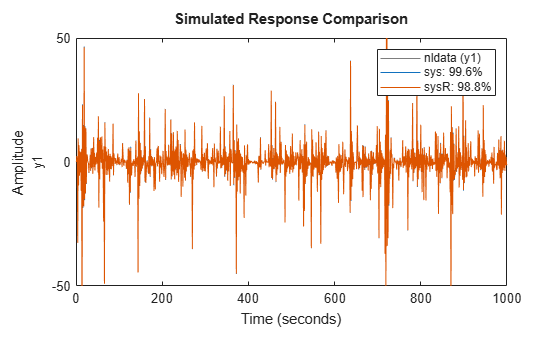The large negative fit result for the unregularized model indicates a poor fit to the data. Estimating a regularized model produces a significantly better result.

## Input Arguments

collapse all

Time-domain estimation data, specified as an `iddata` object or a numeric matrix.

• If `data` is an `iddata` object, then `data` can have one or more output channels and zero or more input channels.

• If `data` is a numeric matrix, then the number of columns of data must match the sum of the number of inputs (nu) and the number of outputs ( ny).

`data` must be uniformly sampled and cannot contain missing (`NaN`) samples.

ARX model orders, specified as the matrix `[na nb nk]`. `na` denotes the number of delayed outputs, `nb` denotes the number of delayed inputs, and `nk` denotes the minimum input delay. The minimum output delay is fixed to `1`. For more information on how to construct the `orders` matrix, see `arx`.

When you specify `orders`, the software converts the order information into linear regressor form in the `idnlarx` `Regressors` property. For an example, see Create Nonlinear ARX Model Using ARX Model Orders.

Regressor specification, specified as a column vector containing one or more regressor specification objects, which are the `linearRegressor` objects, `polynomialRegressor` objects, `periodicRegressor` objects, and `customRegressor` objects. Each object specifies a formula for generating regressors from lagged variables. For example:

• `L = linearRegressor({'y1','u1'},{1,[2 5]})` generates the regressors y1(t–1), u1(t–2), and u2(t–5).

• `P = polynomialRegressor('y2',4:7,2)` generates the regressors y2(t–4)2, y2(t–5)2,y2(t–6)2, and y2(t–7)2.

• `SC = periodicRegressor({'y1','u1'},{1,2})` generates the regressors y1(t-1)), cos(y1(t-1)), sin(u1(t-2)), and cos(u1(t-2)).

• ```C = customRegressor({'y1','u1','u2'},{1 2 2},@(x,y,z)sin(x.*y+z))``` generates the single regressor sin(y1(t–1)u1(t–2)+u2(t–2)

.

When you create a regressor set to support estimation with an `iddata` object, you can use the input and output names of the object rather than create the names for the regressor function. For instance, suppose you create a linear regressor for a model, plan to use the `iddata` object `z` to estimate the model. You can use the following command to create the linear regressor.

`L = linearRegressor([z.outputName;z.inputName],{1,[2 5]})`

For an example of creating and using a SISO linear regressor set, see Estimate Nonlinear ARX Model Using Linear Regressor Set. For an example of creating a MIMO linear regressor set that obtains variable names from the estimation data set, see Estimate MIMO Nonlinear ARX Model with Same Mapping Function for All Outputs.

Output function that maps the regressors of the `idnlarx` model into the model output, specified as a column array containing zero or more of the following strings or objects:

 `'idWaveletNetwork'` or `idWaveletNetwork` object Wavelet network `'linear'` or `''` or `[]` or `idLinear` object Linear function `'idSigmoidNetwork'` or `idSigmoidNetwork` object Sigmoid network `'idTreePartition'` or `idTreePartition` object Binary tree partition regression model `'idGaussianProcess'` or `idGaussianProcess` object Gaussian process regression model (requires Statistics and Machine Learning Toolbox™) `'idTreeEnsemble'` or `idTreeEnsemble` Regression tree ensemble model requires (Statistics and Machine Learning Toolbox) `'idSupportVectorMachine'` or `idSupportVectorMachine` Kernel-based Support Vector Machine (SVM) regression model with constraints (requires Statistics and Machine Learning Toolbox) `idFeedforwardNetwork` object Neural network — Feedforward network of Deep Learning Toolbox™. `idCustomNetwork` object Custom network — Similar to `idSigmoidNetwork`, but with a user-defined replacement for the sigmoid function.

Use a string, such as `'idSigmoidNetwork'`, to use the default properties of the mapping function object. Use the object itself, such as `idSigmoidNetwork`, when you want to configure the properties of the mapping object.

The `idWaveletNetwork`, `idSigmoidNetwork`, `idTreePartition`, and `idCustomNetwork` objects contain both linear and nonlinear components. You can remove (not use) the linear components of `idWaveletNetwork`, `idSigmoidNetwork`, and `idCustomNetwork` by setting the `LinearFcn.Use` value to `false`.

The `idFeedforwardNetwork` function has only a nonlinear component, which is the `network` (Deep Learning Toolbox) object of Deep Learning Toolbox. The `idLinear` object, as the name implies, has only a linear component.

`output_fcn` is static in that it depends only upon the data values at a specific time, but not directly on time itself. For example, if the output function y(t) is equal to y0 + a1 y(t–1) + a2 y(t–2) + … b1 u(t–1) + b2 u(t–2) + …, then `output_fcn` is a linear function that the `linear` mapping object represents.

Specifying a character vector, for example `'idSigmoidNetwork'`, creates a mapping object with default settings. Alternatively, you can specify mapping object properties in two ways:

• Create the mapping object using arguments to modify default properties.

`MO = idSigmoidNetwork(15);`
• Create a default mapping object first and then use dot notation to modify properties.

```MO = idSigmoidNetwork; MO.NumberOfUnits = 15;```

For ny output channels, you can specify mapping objects individually for each channel by setting `output_fcn` to an array of ny mapping objects. For example, the following code specifies `OutputFcn` using dot notation for a system with two input channels and two output channels.

```sys = idnlarx({'y1','y2'},{'u1','u2'}); sys.OutputFcn = [idWaveletNetwork; idSigmoidNetwork];```
To specify the same mapping for all outputs, specify `OutputFcn` as a character vector or a single mapping object.

`output_fcn` represents a static mapping function that transforms the regressors of the nonlinear ARX model into the model output. `output_fcn` is static because it does not depend on time. For example, if $y\left(t\right)={y}_{0}+{a}_{1}y\left(t-1\right)+{a}_{2}y\left(t-2\right)+\dots +{b}_{1}u\left(t-1\right)+{b}_{2}u\left(t-2\right)+\dots$, then `output_fcn` is a linear function represented by the `idLinear` object.

For an example of specifying the output function, see Specify and Customize Output Function.

Discrete-time identified input/output linear model, specified as any linear model created using an estimator such as `arx`, `armax`, `tfest`, or `ssest`. For example, to create a state-space `idss` model, estimate the model using `ssest`.

Nonlinear ARX model, specified as an `idnlarx` model. `sys0` can be:

• A model previously estimated using `nlarx`. The estimation algorithm uses the parameters of `sys0` as initial guesses. In this case, use `init` to slightly perturb the model properties to avoid trapping the model in local minima.

```sys = init(sys); sys = nlarx(data,sys);```
• A model previously created using the `idnlarx` constructor and with properties set using dot notation. For example, use the following to create an idnlarx object, set its properties, and estimate the model.

```sys1 = idnlarx('y1','u1',Regressors); sys1.OutputFcn = 'idTreePartition'; sys1.Ts = 0.02; sys1.TimeUnit = 'Minutes'; sys1.InputName = 'My Data'; sys2 = nlarx(data,sys1);```

The preceding code is equivalent to the following nlarx command.

```sys2 = nlarx(data,Regressors,'idTreePartition','Ts',0.02,'TimeUnit','Minutes', ... 'InputName','My Data');```

Estimation options for nonlinear ARX model identification, specified as an `nlarxOptions` option set. Available options include:

• Minimization objective

• Normalization options

• Regularization options

## Output Arguments

collapse all

Nonlinear ARX model that fits the given estimation data, returned as an `idnlarx` object. This model is created using the specified model orders, nonlinearity estimator, and estimation options.

Information about the estimation results and options used is stored in the `Report` property of the model. The contents of `Report` depend upon the choice of nonlinearity and estimation focus you specified for `nlarx`. `Report` has the following fields:

Report FieldDescription
`Status`

Summary of the model status, which indicates whether the model was created by construction or obtained by estimation.

`Method`

Estimation command used.

`Fit`

Quantitative assessment of the estimation, returned as a structure. See Loss Function and Model Quality Metrics for more information on these quality metrics. The structure has the following fields:

FieldDescription
`FitPercent`

Normalized root mean squared error (NRMSE) measure of how well the response of the model fits the estimation data, expressed as the percentage fitpercent = 100(1-NRMSE).

`LossFcn`

Value of the loss function when the estimation completes.

`MSE`

Mean squared error (MSE) measure of how well the response of the model fits the estimation data.

`FPE`

Final prediction error for the model.

`AIC`

Raw Akaike Information Criteria (AIC) measure of model quality.

`AICc`

Small-sample-size corrected AIC.

`nAIC`

Normalized AIC.

`BIC`

Bayesian Information Criteria (BIC).

`Parameters`

Estimated values of model parameters.

`OptionsUsed`

Option set used for estimation. If no custom options were configured, this is a set of default options. See `nlarxOptions` for more information.

`RandState`

State of the random number stream at the start of estimation. Empty, `[]`, if randomization was not used during estimation. For more information, see `rng`.

`DataUsed`

Attributes of the data used for estimation, returned as a structure with the following fields.

FieldDescription
`Name`

Name of the data set.

`Type`

Data type.

`Length`

Number of data samples.

`Ts`

Sample time.

`InterSample`

Input intersample behavior, returned as one of the following values:

• `'zoh'` — Zero-order hold maintains a piecewise-constant input signal between samples.

• `'foh'` — First-order hold maintains a piecewise-linear input signal between samples.

• `'bl'` — Band-limited behavior specifies that the continuous-time input signal has zero power above the Nyquist frequency.

`InputOffset`

Offset removed from time-domain input data during estimation. For nonlinear models, it is `[]`.

`OutputOffset`

Offset removed from time-domain output data during estimation. For nonlinear models, it is `[]`.

`Termination`

Termination conditions for the iterative search used for prediction error minimization, returned as a structure with the following fields:

FieldDescription
`WhyStop`

Reason for terminating the numerical search.

`Iterations`

Number of search iterations performed by the estimation algorithm.

`FirstOrderOptimality`

$\infty$-norm of the gradient search vector when the search algorithm terminates.

`FcnCount`

Number of times the objective function was called.

`UpdateNorm`

Norm of the gradient search vector in the last iteration. Omitted when the search method is `'lsqnonlin'` or `'fmincon'`.

`LastImprovement`

Criterion improvement in the last iteration, expressed as a percentage. Omitted when the search method is `'lsqnonlin'` or `'fmincon'`.

`Algorithm`

Algorithm used by `'lsqnonlin'` or `'fmincon'` search method. Omitted when other search methods are used.

For estimation methods that do not require numerical search optimization, the `Termination` field is omitted.

For more information on using `Report`, see Estimation Report.

## Algorithms

collapse all

### Nonlinear ARX Model Structure

A nonlinear ARX model consists of model regressors and an output function. The output function contains one or more mapping objects, one for each model output. Each mapping object can include a linear and a nonlinear function that act on the model regressors to give the model output and a fixed offset for that output. This block diagram represents the structure of a single-output nonlinear ARX model in a simulation scenario.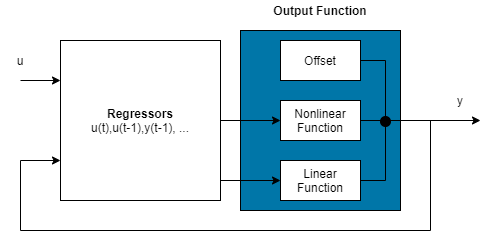The software computes the nonlinear ARX model output y in two stages:

1. It computes regressor values from the current and past input values and the past output data.

In the simplest case, regressors are delayed inputs and outputs, such as u(t–1) and y(t–3). These kind of regressors are called linear regressors. You specify linear regressors using the `linearRegressor` object. You can also specify linear regressors by using linear ARX model orders as an input argument. For more information, see Nonlinear ARX Model Orders and Delay. However, this second approach constrains your regressor set to linear regressors with consecutive delays. To create polynomial regressors, use the `polynomialRegressor` object. To create periodic regressors that contain the sine and cosine functions of delayed input and output variables , use the `periodicRegressor` object. You can also specify custom regressors, which are nonlinear functions of delayed inputs and outputs. For example, u(t–1)y(t–3) is a custom regressor that multiplies instances of input and output together. Specify custom regressors using the `customRegressor` object.

You can assign any of the regressors as inputs to the linear function block of the output function, the nonlinear function block, or both.

2. It maps the regressors to the model output using an output function block. The output function block can include multiple mapping objectslinear, nonlinear, and offset blocks in parallel. For example, consider the following equation:

`$F\left(x\right)={L}^{T}\left(x-r\right)+g\left(Q\left(x-r\right)\right)+d$`

Here, x is a vector of the regressors, and r is the mean of x. $F\left(x\right)={L}^{T}\left(x-r\right)+{y}_{0}$ is the output of the linear function block. $g\left(Q\left(x-r\right)\right)+{y}_{0}$ represents the output of the nonlinear function block. Q is a projection matrix that makes the calculations well-conditioned. d is a scalar offset that is added to the combined outputs of the linear and nonlinear blocks. The exact form of F(x) depends on your choice of output function. You can select from the available mapping objects, such as tree-partition networks, wavelet networks, and multilayer neural networks. You can also exclude either the linear or the nonlinear function block from the output function.

When estimating a nonlinear ARX model, the software computes the model parameter values, such as L, r, d, Q, and other parameters specifying g.

The resulting nonlinear ARX models are `idnlarx` objects that store all model data, including model regressors and parameters of the output function. For more information about these objects, see Nonlinear Model Structures.

## Version History

Introduced in R2007a

expand all

Not recommended starting in R2021b

Not recommended starting in R2021a